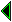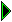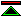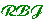# The Root Logic---Some Technical Details

An important early technical step will be to `get off the ground', logically speaking, which we will do by rooting the QED system in a `root logic', whose description requires only a few pages of typical logico-mathematical text. As a model for brevity and clarity, we can refer the reader to Godel's presentation, in about two pages, of high-order logic with number theory and set theory, at the beginning of his famous paper on undecidable questions.

The reason that we emphasize succinctness in the description of the logic is that we hope that there will be many separate implementations of a proof checker for this `root logic' and that each of these implementations can check the correctness of the entire QED system. In the end, it will be the `social process' of mathematical agreement that will lead to confidence in the implementations of these proof-checkers for the root logic of the QED system, and multiple implementations of a succinct logic will greatly increase the chance this social process will occur.

It is crucial that a `root logic' be a logic that is agreeable to all practicing mathematicians. The logic will, by necessity, be sufficiently strong to check any explicit computation, but the logic surely must not prejudge any historically debated questions such as the law of the excluded middle or the existence of uncountable sets.

As just one hint of a logic that might be used as the basis of QED, we mention Primitive Recursive Arithmetic (PRA) which is the logic Skolem invented for the foundations of arithmetic, which was later adopted by Hilbert-Bernays as the right vehicle for proof theory. It has also been further developed by Goodstein. In PRA one finds (a) an absence of explicit quantification, (b) an ability to define primitive recursive functions, (c) a few rules for handling equality, e.g., substitution of equals for equals, (d) a rule of instantiation, and (e) a simple induction principle. One reason for taking such a logic as the root logic is that it is doubtful that Metamathematics can be developed in a weaker logic. In any root logic one needs to be able to define, inductively, an infinite collection of terms and, inductively, an infinite collection of theorems, using in the definition of `theorem' such primitive recursive concepts as substitution. Thus PRA has the bare minimum power we would need to `get off the ground'. Yet we think it suffices even for checking theorems in classical set theory, in a sense we describe below. The logic FS0, conservative over PRA, but with sets and quantifiers, has been proposed by Feferman as a vehicle more congenial than PRA for studying logics.

It is probably the case that the syntax of resolution theorem-proving is the most widely used and most easily understood logic in the history of work on mechanical theorem-proving and proof checking, and thus perhaps a resolution-like logic could serve as a natural choice for a root logic. Some may object on the grounds that resolution, being based upon classical first order logic, ``wires in'' the law of the excluded middle, and therefore is objectionable to constructivists. In response to this objection, let us note that constructivists do not object to the law of the excluded middle in a free variable setting if all of the predicates and function symbols ``in sight'' are recursively defined; for example, it is a constructive theorem that for all positive integers x and y, x divides y or x does not divide y. Thus we might imagine taking as a root logic resolution restricted to axioms describing recursive functions and hereditarily finite objects, such as the integers.

The lambda-calculus-based ``logical frameworks'' work in Europe, in the de Bruijn tradition, is perhaps the most well developed potential root logic, with several substantial computer implementations which have already checked significant parts of mathematics. And already, many different logics have been represented in these logical frameworks. As a caution, we note that some may worry there is dangerously too much logical power in some of these versions of the typed lambda calculus. But such logical frameworks give rise to the hope that the root logic might be such that classical logic could simply be viewed as the extension of the root logic by a few higher-order axioms such as (all P) (Or P (Not P)).

One possible argument in favor of adopting a root logic of power PRA is that its inductive power permits the proof of metatheorems, which will enable the QED system to check and then effectively use decision procedures. For example, the deduction theorem for first order logic is a theorem of FS0, something not provable in some logical framework systems, for want of induction.

Regardless of the strength or weakness of the root logic chosen, we believe that we can rigorously incorporate into the QED system any part of mathematics, including extremely non-constructive set theoretic arguments, because we can represent these arguments `one level removed' as `theorems' that a certain finite object is indeed a proof in a certain theory. For example, if we have in mind some high powered theorem, say, the independence of the continuum hypothesis, we can immediately think of a corresponding theorem of primitive recursive arithmetic that says, roughly, that some sequence of formulas is a proof in some suitable set theory, S1, of another theorem about some other set theory, where a, say, primitive recursive proof checker for S1 has been written in the root logic of QED. In practice, it will be highly advantageous if we make it appear that one isn't really proving a theorem of proof theory but rather is proving a theorem of group theory or topology or whatever.

Although many groups have built remarkable theorem-proving and proof checking systems, we believe that there is a need for some further scientific or computational advances to overcome some `resource' problems in building a system that can hold all important mathematics. Simply stated, it appears that complete proofs of certain theorems that involve a lot of computation will require more disk space for their storage than could reasonably be expected to be available for the project. The most attractive solution to such a problem is the development of `reflection' techniques that will permit one to use algorithms that have been rigorously incorporated within QED as part of the QED proof system.

Although we have spoken of a single root logic, we need to make clear that we do not want to fall into the trap of searching for a single, ideal logic. We can easily imagine that it will be possible to develop several different root logics each of which can be fully regarded to be `a' foundation of QED, each of which is capable as acting as a basis for the other, and each of which has very short implementations which have been checked by the `social process'. And each of which can be used to check the correctness of the entire QED system.

In any case, it is a highly desireable goal that a checker for the root logic can be easily written in common programming languages. The specification should be so unambiguous that many can easily implement it from its specification in a few pages of code, with total comprehension by a single person.

It has been argued that the idea of having multiple logics in addition to the root logic is a mistake that will result in too much complexity, and that it would be far more sensible to have a single logic in which proofs were clearly flagged with an indication of the assumptions used, so that a single logic could be enjoyed by people of both classical and constructive persuasions. Certainly such a single logic is desireable, but whether such a single logic can be developed is a serious question given that some famous constructive theorems (such as the continuity of all functions on the reals) are classical falsehoods.

It has been argued that the idea of searching for a single logic or a single computer system is inferior to the idea of developing translation mechanisms that would permit proof checking systems to exchange proofs with one another. If this were feasible, it would certainly permit an alternative, distributed approach to achieving the major QED objectives. However, the history of radical incompatibility of many proof checking systems does suggest that such translation mechanisms may be difficult to produce.

In seeking a root logic, it is clear that there will be many controversies that will be impossible to resolve to everyone's satisfaction. For example, there seems no hope of satisfying in a single logic those who insist upon a typed syntax and those who loathe typed syntax, preferring to do typing internally, e.g., with sets. There are also simple questions not yet resolved after centuries of thought, such as the semantics of a function applied outside its domain, e.g., division by zero.created 31/12/95 modified 11/1/96